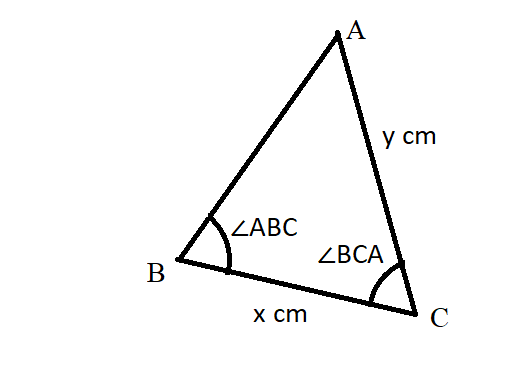# Suppose that m /_BCA=70^@, and x=33 cm and y = 47 cm. What is the degree measure of /_ABC?01510101651.pngReggie 2020-12-27 Answered

Suppose that $m\mathrm{\angle }BCA={70}^{\circ }$, and $x=33$ cm and $y=47$ cm. What is the degree measure of $\mathrm{\angle }ABC$?You can still ask an expert for help

• Questions are typically answered in as fast as 30 minutes

Solve your problem for the price of one coffee

• Math expert for every subject
• Pay only if we can solve itBrittany Patton
$AB=\sqrt{A{C}^{2}+B{C}^{2}-\left(2\cdot AC\cdot BC\mathrm{cos}\mathrm{\angle }BCA\right)}$
$AB=\sqrt{{47}^{2}+{33}^{2}-\left(2\cdot 47\cdot 33{\mathrm{cos}70}^{\circ }\right)}$
$AB=\sqrt{2237.05}$
$AB=47.29$
Using the cosine law,
$\mathrm{\angle }ABC={\mathrm{cos}}^{-1}\left(\frac{B{C}^{2}+A{B}^{2}-A{C}^{2}}{2\cdot BC\cdot AB}\right)$
$\mathrm{\angle }ABC={\mathrm{cos}}^{-1}\left(\frac{{33}^{2}+{47.29}^{2}-{47}^{2}}{2\cdot 33\cdot 47.29}\right)$
$\mathrm{\angle }ABC={\mathrm{cos}}^{-1}\left(0.3576\right)$
$\mathrm{\angle }ABC={\mathrm{cos}}^{-1}={69.04}^{\circ }$
###### Not exactly what you’re looking for?

Step 1

Given that

x=33 cm, y=47 cm

$m\mathrm{\angle }BCA={70}^{\circ }$

$\mathrm{\angle }ABC=?$

using cosine Rules

$\mathrm{cos}C=\frac{\left(AC{\right)}^{2}+\left(BC{\right)}^{2}-\left(AB{\right)}^{2}}{2\left(AC\right)\left(BC\right)}$

$\mathrm{cos}{70}^{\circ }=\frac{\left(47{\right)}^{2}+\left(33{\right)}^{2}-\left(AB{\right)}^{2}}{2×47×33}$

$0.3420=\frac{3298-\left(AB{\right)}^{2}}{3102}$

AB=47.30 cm

Using sine rule

$\frac{\mathrm{sin}\mathrm{\angle }ABC}{47}=\frac{\mathrm{sin}\mathrm{\angle }BCA}{47.30}$

$\mathrm{sin}\mathrm{\angle }ABC=\frac{47}{47.30}×\mathrm{sin}{70}^{\circ }$

$\mathrm{sin}\mathrm{\angle }ABC=0.9337$

$\mathrm{\angle }ABC\approx {68.24}^{\circ }$

$\mathrm{\angle }ABC\approx {68.72}^{\circ }$